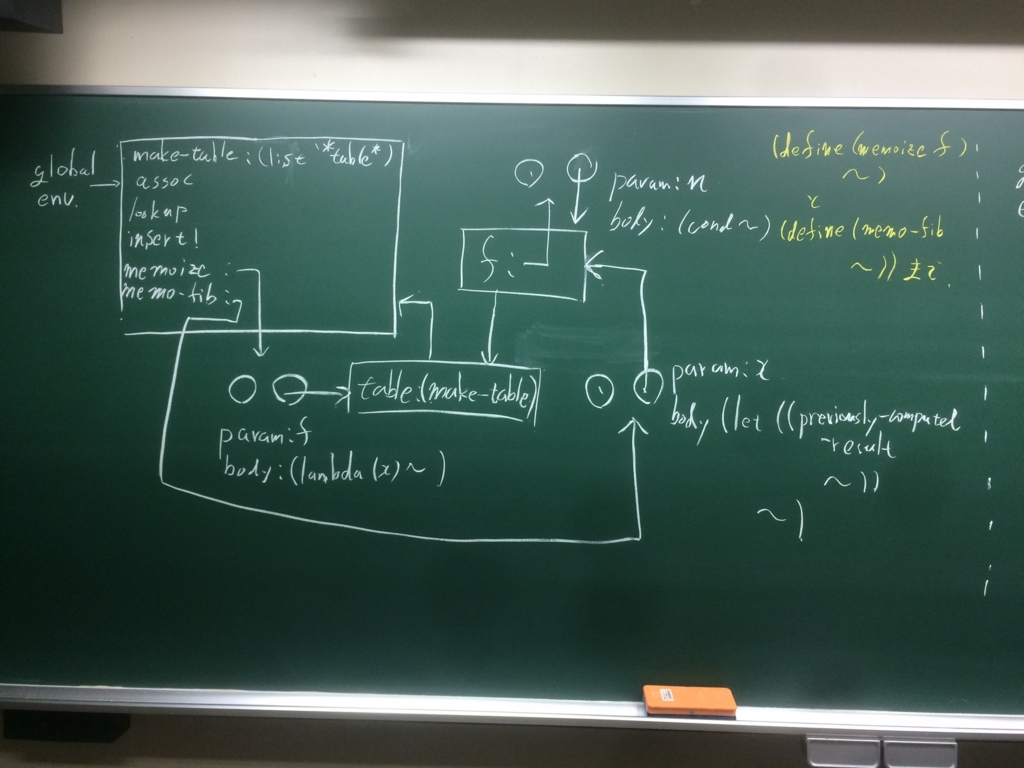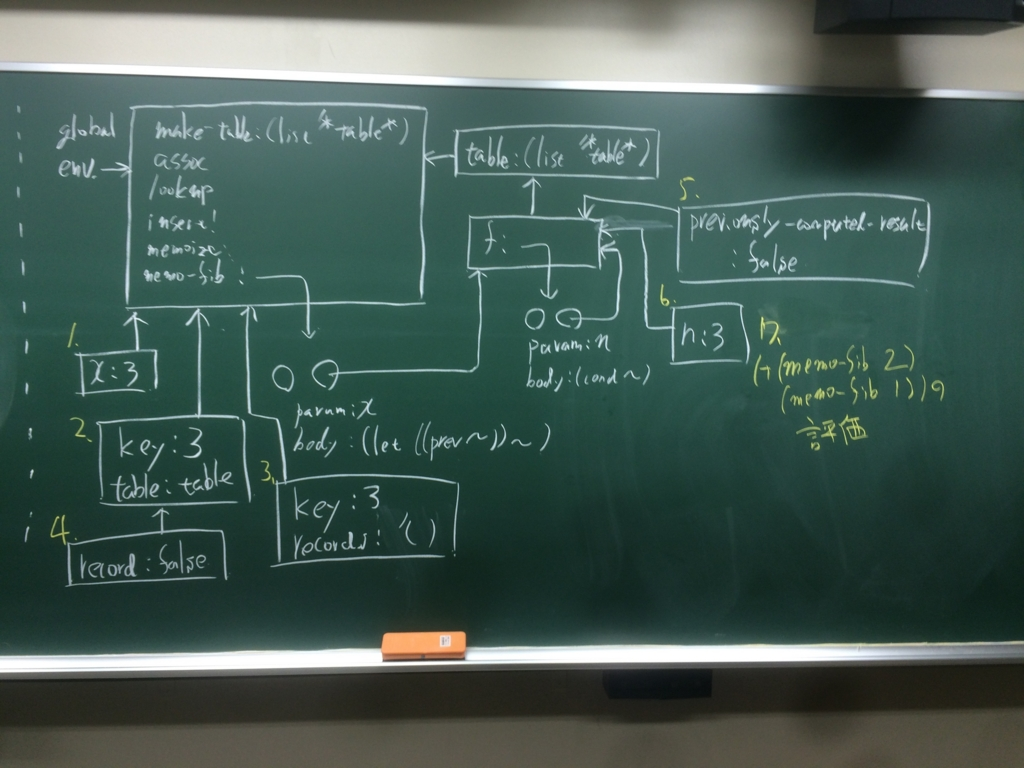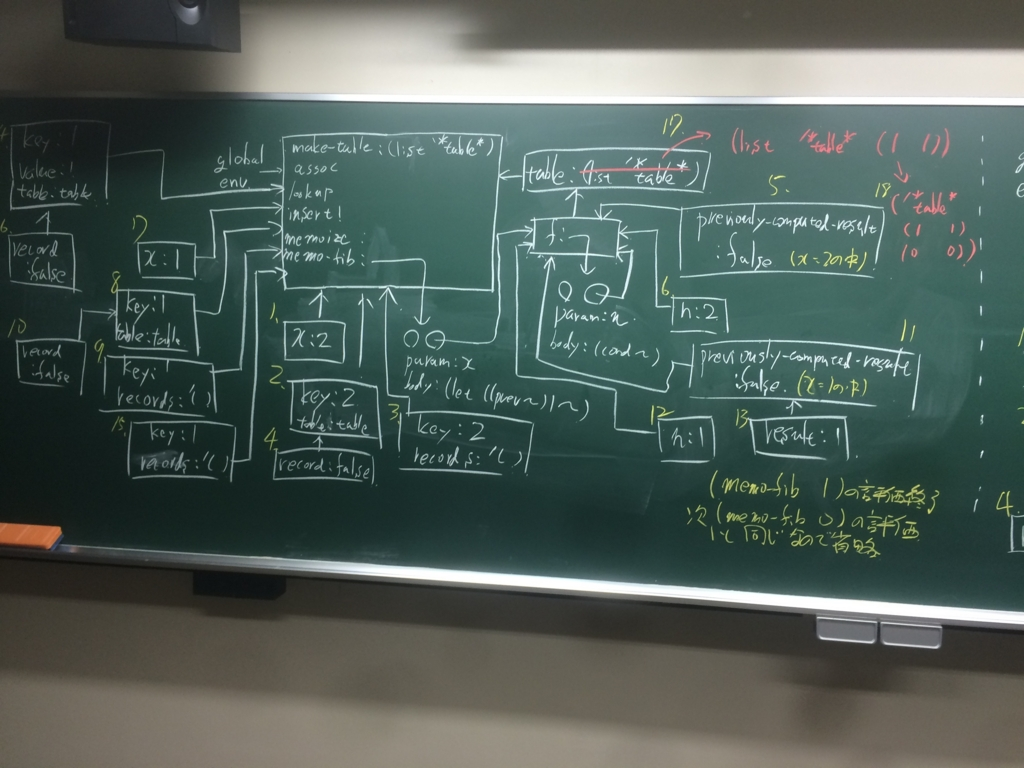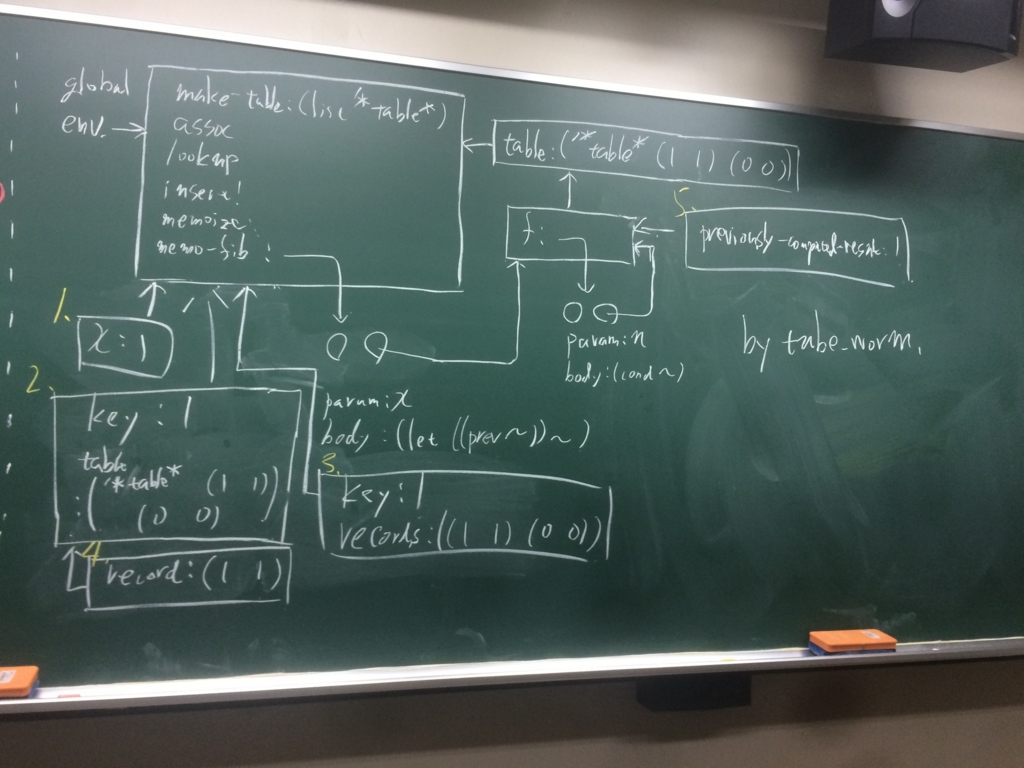SICPゼミ第20回

練習問題3.27練習問題3.28
```(define (or-gate a1 a2 output)
(define (or-action-procedure)
(let ((new-value (logical-or (get-signal a1) (get-signal a2))))
(after-delay or-gate-delay (lambda () (set-signal! output new-value)))))
'ok)

(define (logical-or s1 s2)
(if (or (= s1 1) (= s2 1)) 1 0))
```

by pine

練習問題3.29
```(define (or-gate2 a1 a2 output)
(define w1 (make-wire))
(define w2 (make-wire))
(define w3 (make-wire))
(inverter a1 w1)
(inverter a2 w2)
(and-gate w1 w2 w3)
(inverter w3 output))
```

by pine

練習問題3.30
```(define (ripple-carry-adder n A B S)
(define (ripple-carry-adder-iter n m A B S C)
(let ((c (make-wire)))
(begin (full-adder (car A) (car B) C (car S) c)
(+ m 1)
(if (= n m)
'done
(ripple-carry-adder-iter n m (cdr A) (cdr B) (cdr S) c)
)
)
)
)
(begin (define c (make-wire)) (half-adder (car A) (car B) (car S) c)
(ripple-carry-adder-iter n 1 (cdr A) (cdr B) (cdr S) c)
)
)
```